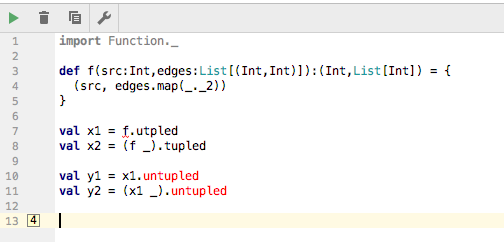# How to map an n-arity function to 1-arity function of tuple

If I have a function one some arity, say `n`, and I want to convert it to a unary function, what is the best way to do it? Do I need to write it by hand every time, or is there an operator I can use?

For example, suppose I have a function like,

``````def f(x1:A1, x2: A2, ... xn:An):B = {
...
}
``````

and I need to give this function to a `map`, `hashMap`, `fold` etc, which is expecting a tuple of type `((A1,A2,...An)) => B`

Is there a function such as `n-arity-function-to-appropriate-tuple-function` ?

I think I’ve almost found the answer. From tupled/untupled

I can’t use the syntax `f.tupled`, but I can use `(f _).tupled`.
However, I cannot figure out how to use `untupled` at all.

``````import Function._

def f(src:Int,edges:List[(Int,Int)]):(Int,List[Int]) = {
(src, edges.map(_._2))
}

val x1 = f.utpled
val x2 = (f _).tupled

val y1 = x1.untupled
val y2 = (x1 _).untupled
``````

Which is colorized in IntelliJ as follows.You can use `Function.tupled(f _)` and `Function.untupled(f _)`.

How is `Function.tupled(f _)` different from `(f _).tupled` ?

In the first, `tupled` is a method defined on the object `Function`. In the second, `tupled` is a method defined on a trait `FunctionN` where `N` is equal to the arity of method `f`.

For `tupled` it doesn’t really make a difference, but `untupled` only exists in the `Function` object.

1 Like

Interesting error when I true to use it in its syntactically correct form.

``````import Function._

def f(src:Int,edges:List[(Int,Int)]):(Int,List[Int]) = {
(src, edges.map(_._2))
}
val x1 = tupled(f _)
val y1 = untupled(x1 _)
``````
``````Error:(7, 18) overloaded method value untupled with alternatives:
[a1, a2, a3, a4, a5, b](f: ((a1, a2, a3, a4, a5)) => b)(a1, a2, a3, a4, a5) => b <and>
[a1, a2, a3, a4, b](f: ((a1, a2, a3, a4)) => b)(a1, a2, a3, a4) => b <and>
[a1, a2, a3, b](f: ((a1, a2, a3)) => b)(a1, a2, a3) => b <and>
[a1, a2, b](f: ((a1, a2)) => b)(a1, a2) => b
cannot be applied to (() => ((Int, List[(Int, Int)])) => (Int, List[Int]))
val y1 = untupled(x1 _)
``````

I think `untupled(x1)` should work. `x1` is already a function object.

1 Like

To be precise, here `f` is a method, not a function. Syntax construct `f _` in turn is described as lifting method `f` to a function.

Just to recap a couple of things of interest here, you must keep in mind that

• methods and functions are not the same in scala
• a method is defined on an object instance
• a function is an object in his own right with a specific method called apply, that allow you to… “apply the function” to some arguments, using a shorthand syntax that makes its use similar to methods

This subtle difference gives rise to a lot of puzzling behaviour, as most function operators are not valid for methods. That’s when the `f _` syntax is used to “lift the method as a function object”.

Further confusion is due to the fact that there exists usages when the lifting is implicitly done by the compiler, if the context makes it clear that you expected a function and a method with matching parameters is passed (e.g. higher order functions like `map, fold`)

You can find additional information in this useful article

1 Like